In the last tutorial we learned how to create and move simple shapes with signed distance functions. In this one we will learn how to combine several shapes to make more complex distance fields. I learned most of the techniques described here from a glsl signed distance function library you can find here (http://mercury.sexy/hg_sdf) and there are a few ways of combining shapes I don’t go into here.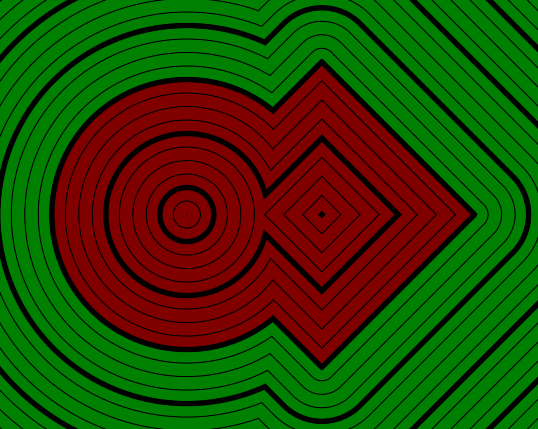## Setup

To visualise the signed distance fields, we’re going to make one basic setup and then use the operators with it. It will use the distance lines visualisation we made in the first tutorial for showing the distance fields. We will set all parameters except for the visualisation parameters in code for simplicity, but you can replace any value you see with a property to make it adjustable.

The main shader we start with looks like this:

``````Shader "Tutorial/035_2D_SDF_Combinations/Champfer Union"{
Properties{
_InsideColor("Inside Color", Color) = (.5, 0, 0, 1)
_OutsideColor("Outside Color", Color) = (0, .5, 0, 1)

_LineDistance("Mayor Line Distance", Range(0, 2)) = 1
_LineThickness("Mayor Line Thickness", Range(0, 0.1)) = 0.05

[IntRange]_SubLines("Lines between major lines", Range(1, 10)) = 4
_SubLineThickness("Thickness of inbetween lines", Range(0, 0.05)) = 0.01
}

SubShader{
//the material is completely non-transparent and is rendered at the same time as the other opaque geometry
Tags{ "RenderType"="Opaque" "Queue"="Geometry"}

Pass{
CGPROGRAM

#include "UnityCG.cginc"
#include "2D_SDF.cginc"

#pragma vertex vert
#pragma fragment frag

struct appdata{
float4 vertex : POSITION;
};

struct v2f{
float4 position : SV_POSITION;
float4 worldPos : TEXCOORD0;
};

v2f vert(appdata v){
v2f o;
//calculate the position in clip space to render the object
o.position = UnityObjectToClipPos(v.vertex);
//calculate world position of vertex
o.worldPos = mul(unity_ObjectToWorld, v.vertex);
return o;
}

float scene(float2 position) {
const float PI = 3.14159;

float2 squarePosition = position;
squarePosition = translate(squarePosition, float2(1, 0));
squarePosition = rotate(squarePosition, .125);
float squareShape = rectangle(squarePosition, float2(2, 2));

float2 circlePosition = position;
circlePosition = translate(circlePosition, float2(-1.5, 0));
float circleShape = circle(circlePosition, 2.5);

float combination = combination_function(circleShape, squareShape);

return combination;
}

float4 _InsideColor;
float4 _OutsideColor;

float _LineDistance;
float _LineThickness;

float _SubLines;
float _SubLineThickness;

fixed4 frag(v2f i) : SV_TARGET{
float dist = scene(i.worldPos.xz);
fixed4 col = lerp(_InsideColor, _OutsideColor, step(0, dist));

float distanceChange = fwidth(dist) * 0.5;
float majorLineDistance = abs(frac(dist / _LineDistance + 0.5) - 0.5) * _LineDistance;
float majorLines = smoothstep(_LineThickness - distanceChange, _LineThickness + distanceChange, majorLineDistance);

float distanceBetweenSubLines = _LineDistance / _SubLines;
float subLineDistance = abs(frac(dist / distanceBetweenSubLines + 0.5) - 0.5) * distanceBetweenSubLines;
float subLines = smoothstep(_SubLineThickness - distanceChange, _SubLineThickness + distanceChange, subLineDistance);

return col * majorLines * subLines;
}

ENDCG
}
}
FallBack "Standard" //fallback adds a shadow pass so we get shadows on other objects
}
``````

And the 2D_SDF.cginc function in the same folder as the shader we will expand looks like this at the start:

``````#ifndef SDF_2D
#define SDF_2D

//transforms

float2 rotate(float2 samplePosition, float rotation){
const float PI = 3.14159;
float angle = rotation * PI * 2 * -1;
float sine, cosine;
sincos(angle, sine, cosine);
return float2(cosine * samplePosition.x + sine * samplePosition.y, cosine * samplePosition.y - sine * samplePosition.x);
}

float2 translate(float2 samplePosition, float2 offset){
//move samplepoint in the opposite direction that we want to move shapes in
return samplePosition - offset;
}

float2 scale(float2 samplePosition, float scale){
return samplePosition / scale;
}

//shapes

float circle(float2 samplePosition, float radius){
//get distance from center and grow it according to radius
return length(samplePosition) - radius;
}

float rectangle(float2 samplePosition, float2 halfSize){
float2 componentWiseEdgeDistance = abs(samplePosition) - halfSize;
float outsideDistance = length(max(componentWiseEdgeDistance, 0));
float insideDistance = min(max(componentWiseEdgeDistance.x, componentWiseEdgeDistance.y), 0);
return outsideDistance + insideDistance;
}

#endif
``````

## Simple Combinations

We’ll start with a few simple ways of combining two shapes to make one bigger shape, unions, intersections and subtractions. And a way to make one shape morph into another.

### Union

The most basic operator is a union. With it we can add two shapes together and get the signed distance of the combined shape. When we have the signed distance of two shapes we can combine them by taking the smaller value of the two with the `min` function.

By using the minimum of the two values the end shape will be below 0 (visible) where one of the two input shapes has a distance to the edge which is below 0, the same works for all other distance values, showing the combination of both shapes.

I call the function to create a union “merge” here, partly because it’s the act of merging them, partly because the union keyword is reserverd in hlsl so we can’t use it as a function name.

``````//in 2D_SDF.cginc include file

float merge(float shape1, float shape2){
return min(shape1, shape2);
}
``````
``````//in scene function in shader

float combination = merge(circleShape, squareShape);
``````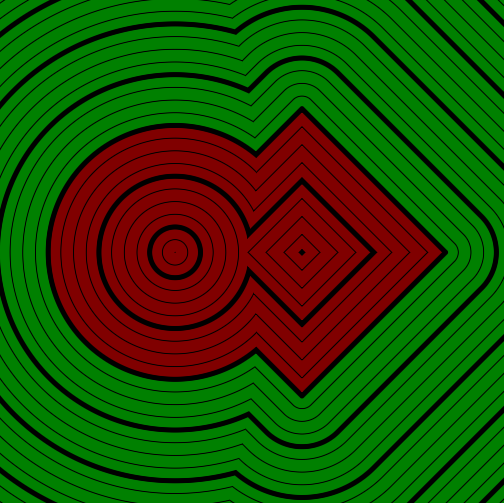### Intersection

Another common way of combining shapes is to use the areas where two shapes overlap. For this we take the maximum value of the two shape distances we want to combine. When using the bigger value of the two, we get a value above 0 (outside the shape) whenever any of the distances to the two shapes is outside the shape and the other distances line up similarly again.

``````//in 2D_SDF.cginc include file

float intersect(float shape1, float shape2){
return max(shape1, shape2);
}
``````
``````//in scene function in shader

float combination = intersect(circleShape, squareShape);
``````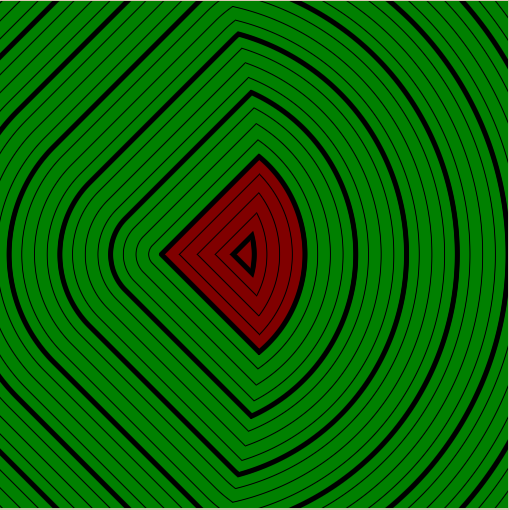### Subtraction

Often we don’t want to treat both shapes the same though, instead we want to subtract one shape from the other. This is pretty easy to do by doing a intersection between the shape we want to modify and everything but the shape we want to subtract. The way we get invert the inside and outside of a shape is by negating the signed distance. What was 1 unit outside the shape is 1 unit inside it now.

``````//in 2D_SDF.cginc include file

float subtract(float base, float subtraction){
return intersect(base, -subtraction);
}
``````
``````//in scene function in shader

float combination = subtract(squareShape, circleShape);
``````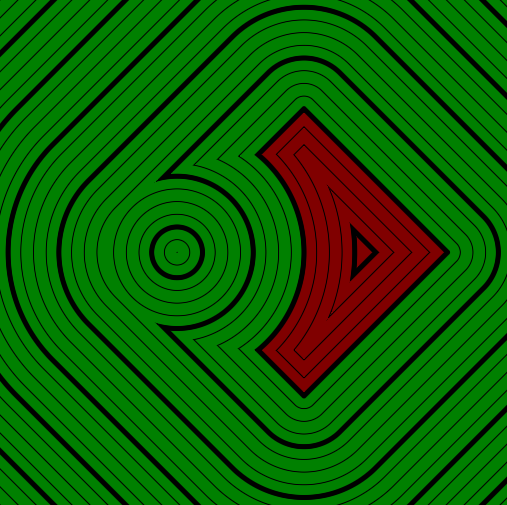### Interpolation

A non-obvious way of combining two shapes is to interpolate beteen them. This is also possible to some extent with polygon meshes with blendshapes, but is way more limited that what we can do with signed distance fields. By simply interpolating between the distances of two shapes they smoothly morph into each other. For the interpolation we can simply use the `lerp` method.

``````//in 2D_SDF.cginc include file

float interpolate(float shape1, float shape2, float amount){
return lerp(shape1, shape2, amount);
}
``````
``````//in scene function in shader

float pulse = sin(_Time.y) * 0.5 + 0.5;
float combination = interpolate(circleShape, pulse);
``````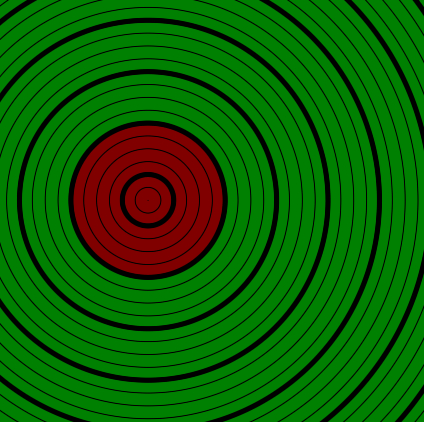## Other Combinations

With the simply combinations we already have everything we need to just combine shapes, but the wonderful thing about signed distance fields is that we aren’t limited to this, there are many other ways we can combine shapes and do interresting stuff where they connect. Again, I’ll only explain a few of the techniques, but you can see more of them at http://mercury.sexy/hg_sdf(write me if you know more useful SDF libraries).

### Round

We can interpret the surface of the two shapes we’re combining as the x and y axis of position in a coordinate system and then calculate the distance to the origin of that position. If we do that it’ll give us a really weird shape, but if we limit the axis to values below 0 we get something that looks like a smooth union of the inside distances of the two shapes.

``````float round_merge(float shape1, float shape2, float radius){
float2 intersectionSpace = float2(shape1, shape2);
intersectionSpace = min(intersectionSpace, 0);
return length(intersectionSpace);
}
``````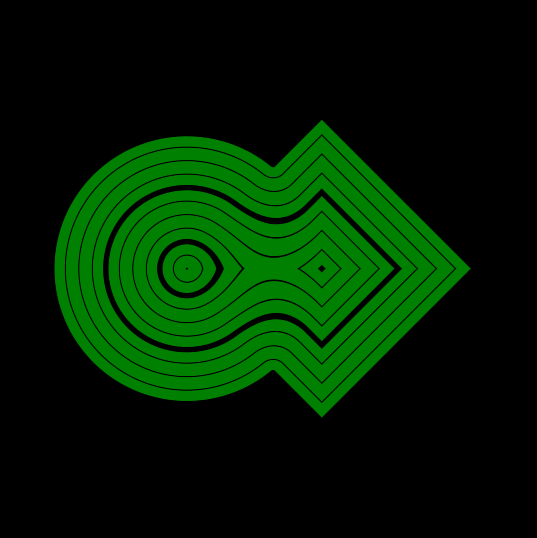That’s nice, but we can’t change the line where the distance is 0 is with this, so it’s not more valuable that a regular union so far. But what we can do is to grow the two shapes bigger before combining them. Similarly to when we created the circle, to grow a shape we subtract from it’s distance to push the line where the signed distance is 0 further outside.

``````float radius = max(sin(_Time.y * 5) * 0.5 + 0.4, 0);
float combination = round_intersect(squareShape, circleShape, radius);
``````
``````float round_merge(float shape1, float shape2, float radius){
float2 intersectionSpace = float2(shape1 - radius, shape2 - radius);
intersectionSpace = min(intersectionSpace, 0);
return length(intersectionSpace);
}
``````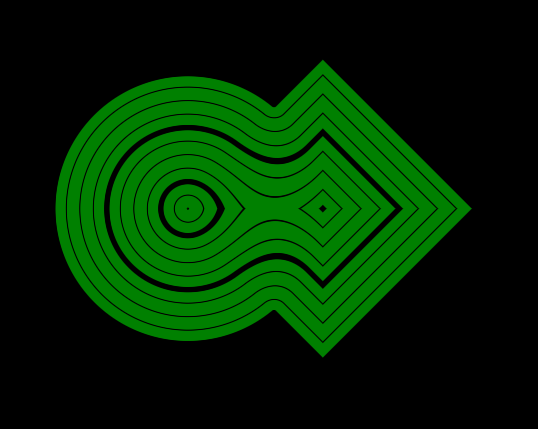This just grows our shape and makes sure the transition inside is smooth, but we don’t want to grow the shapes, we just want a smooth transition. The solution for this is to subtract the radius again after we calculated the length, most parts will look just like before, except the transition between the shapes which is smoothed nicely based on the radius. Just ignore the outside of the shape for now.

``````float round_merge(float shape1, float shape2, float radius){
float2 intersectionSpace = float2(shape1 - radius, shape2 - radius);
intersectionSpace = min(intersectionSpace, 0);
return length(intersectionSpace) - radius;
}
``````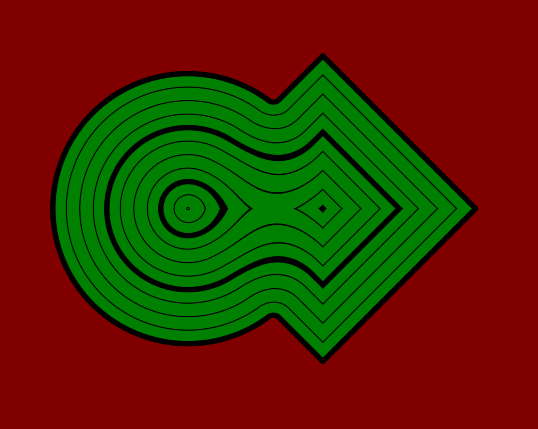The last step is to fix the outside of the shape. Also right now the inside of the shape is green which is the color we use for the outside. The first step is to flip inside and outside by simply inverting the signed distance. Then we replace the part where we subtract the radius. First we change it from a subtraction to a addition. That’s because we invert the distance of the vector before combining it with the radius, so to keep in line with the inversion we also invert the math operation we use. Then we replace the radius with a regular union, this gives us correct values outside of the shape, but not close to the edge or inside it, to avoid that we take the maximum between it and the radius, this way we get the positive of correct values outside of the shape as well as the addition of the radius inside it we need.

``````float round_merge(float shape1, float shape2, float radius){
float2 intersectionSpace = float2(shape1 - radius, shape2 - radius);
intersectionSpace = min(intersectionSpace, 0);
float insideDistance = -length(intersectionSpace);
float simpleUnion = merge(shape1, shape2);
float outsideDistance = max(simpleUnion, radius);
return  insideDistance + outsideDistance;
}
``````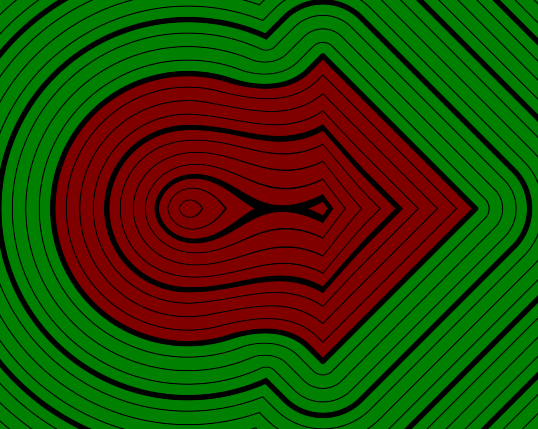For the Intersection we have to do the opposite, we shrink the shapes by the radius, make sure the components of the vector are above 0 and take the length and don’t invert it. This builds the outside of the shape. Then to create the inside we take a regular intersection and make sure it doesn’t get lower than minus the radius. Then we add the inside and outside values just like before.

``````float round_intersect(float shape1, float shape2, float radius){
float2 intersectionSpace = float2(shape1 + radius, shape2 + radius);
intersectionSpace = max(intersectionSpace, 0);
float outsideDistance = length(intersectionSpace);
float simpleIntersection = intersect(shape1, shape2);
float insideDistance = min(simpleIntersection, -radius);
return outsideDistance + insideDistance;
}
``````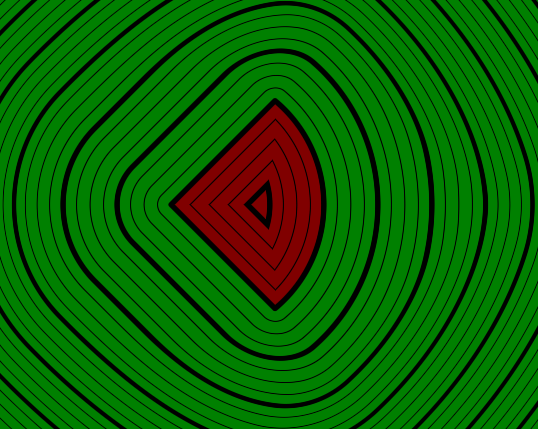And as a last point the subtraction can again be descibed as a intersection between the base shape and everything except the shape we’re subtracting.

``````float round_subtract(float base, float subtraction, float radius){
round_intersect(base, -subtraction, radius);
}
``````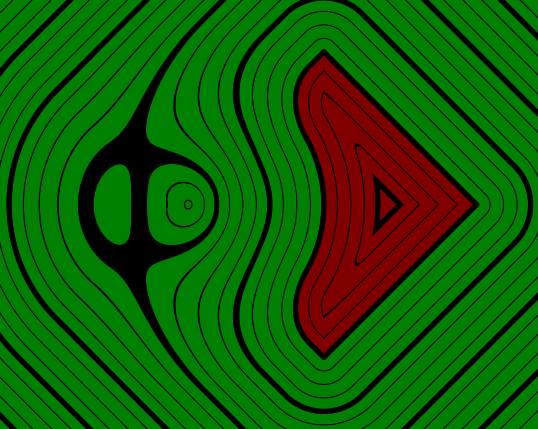Especially in the subtraction you can see the artefacts that come from assuming that we can use the two shapes as coordinates, but the distance field is still good enough to use it for most purposes.

### Champfer

Another thing we can do is to to champfer the transition to give it a bevel like corner. To get that effect we first create a new shape by adding the existing two. If we again assume the point where the two shapes meet is orthogonal this operation would give us a diagonal line which goes though the point where the two surfaces meet.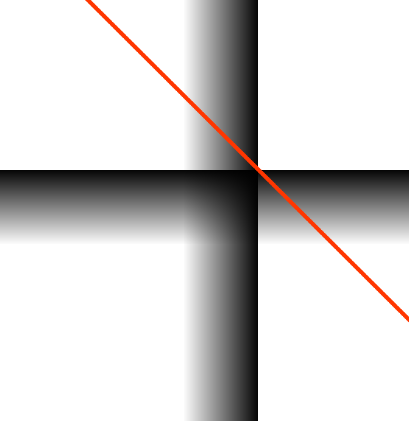because we simply added the two components, the signed distance of this new line has the wrong scaling, but we can fix it by dividing it by the diagonal of a unit square, the square root of 2. Dividing by the square root of 2 is the same as multiplying it with the square root of 0.5 and we can simply write that value into our code to not make it calculate the same root every time.

Now that we have a shape that has the shape of our champfer, we can expand it to make the chamfer be outside the shape. Just like previously, we subtract the amount we want to make the shape expand. Then we merge the champfer shape with the output of a regular merge and we have a champfered transition.

``````float champferSize = sin(_Time.y * 5) * 0.3 + 0.3;
float combination = champfer_merge(circleShape, squareShape, champferSize);
``````
``````float champfer_merge(float shape1, float shape2, float champferSize){
const float SQRT_05 = 0.70710678118;
float simpleMerge = merge(shape1, shape2);
float champfer = (shape1 + shape2) * SQRT_05;
champfer = champfer - champferSize;
return merge(simpleMerge, champfer);
}
``````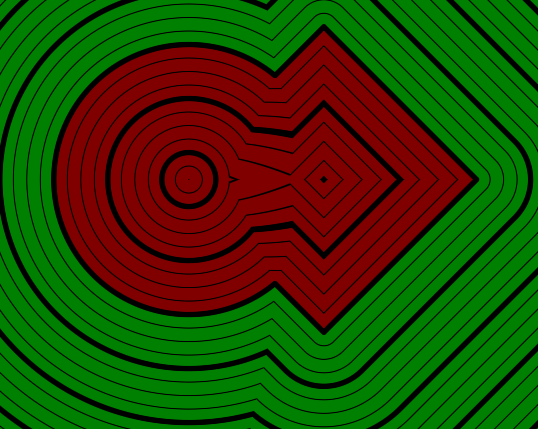For the intersected champfer we add the two shapes like previously, but then we shrink the shape by adding the champfer amount and then doing a intersection with the regular intersected shape.

``````float champfer_intersect(float shape1, float shape2, float champferSize){
const float SQRT_05 = 0.70710678118;
float simpleIntersect = intersect(shape1, shape2);
float champfer = (shape1 + shape2) * SQRT_05;
champfer = champfer + champferSize;
return intersect(simpleIntersect, champfer);
}
``````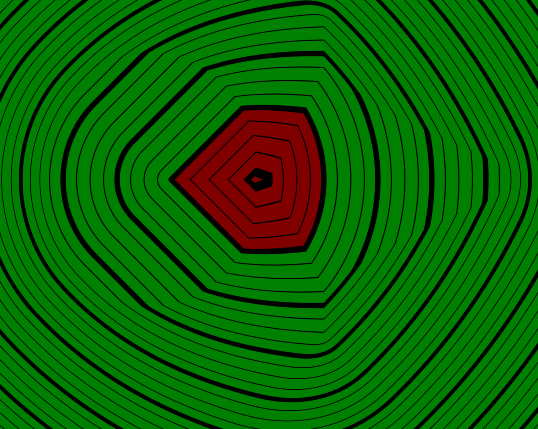And similarly to the previous subtractions we can also do a intersection with a inverted second shape here.

``````float champfer_subtract(float base, float subtraction, float champferSize){
return champfer_intersect(base, -subtraction, champferSize);
}
``````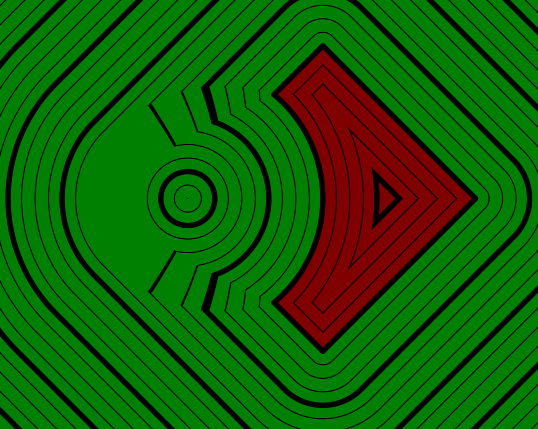### Round Intersection

So far we only used boolean operators (apart from interpolating). But we can also combine the shapes in other ways, for example we can create new round shapes where the borders of two shapes overlap.

To do this we again interpret the two shapes as the x and y axis of a point. Then we simply calculate that points distance from the origin. Where the shapes borders overlap, the distance of both shapes will be 0, giving us a distance of 0 from the origin in our made-up coordinate system. Then that we have the distance from the origin we can give it the same treatment as we did for circles and subtract the radius.

``````float round_border(float shape1, float shape2, float radius){
float2 position = float2(shape1, shape2);
float distanceFromBorderIntersection = length(position);
return distanceFromBorderIntersection - radius;
}
``````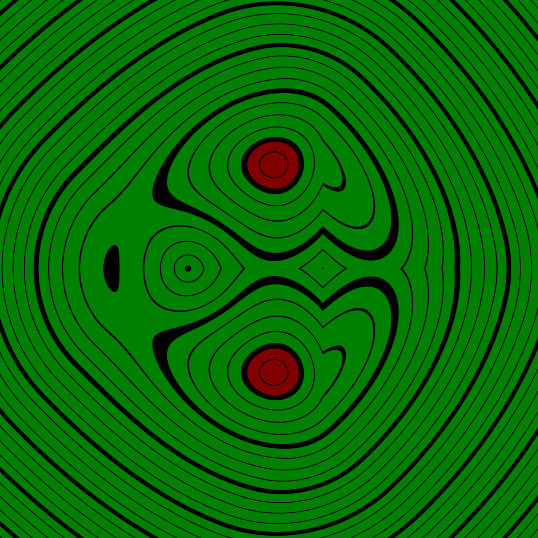### Border Groove

The last technique I’ll explain is a way to make a groove in one shape at the position of the border of another shape.

We start by calculating the shape of the border of the circle. We do this by getting the absolute value of the distance of the first shape, this way inside as well as outside counts as inside the shape, but the border still has the value of 0. If we grow this shape by subtracting the width of the groove we get a shape around the border of the previous shape.

``````float depth = max(sin(_Time.y * 5) * 0.5 + 0.4, 0);
float combination = groove_border(squareShape, circleShape, .3, depth);
``````
``````float groove_border(float base, float groove, float width, float depth){
float circleBorder = abs(groove) - width;
return circleBorder;
}
``````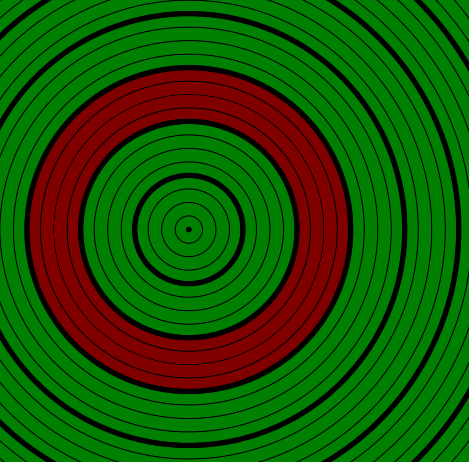Then we want the circle border to only go as deep as we specify. To do this we subtract a shrunk version of the base shape from it. The amount we shrink the base is the depth of the groove.

``````float groove_border(float base, float groove, float width, float depth){
float circleBorder = abs(groove) - width;
float grooveShape = subtract(circleBorder, base + depth);
return grooveShape;
}
``````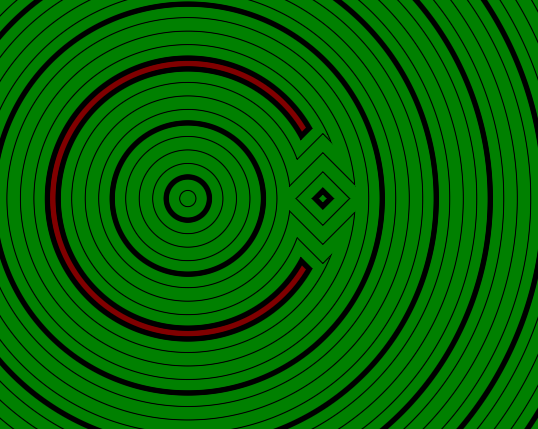Then as a last step we subtract the groove from the base shape and return the result.

``````float groove_border(float base, float groove, float width, float depth){
float circleBorder = abs(groove) - width;
float grooveShape = subtract(circleBorder, base + depth);
return subtract(base, grooveShape);
}
``````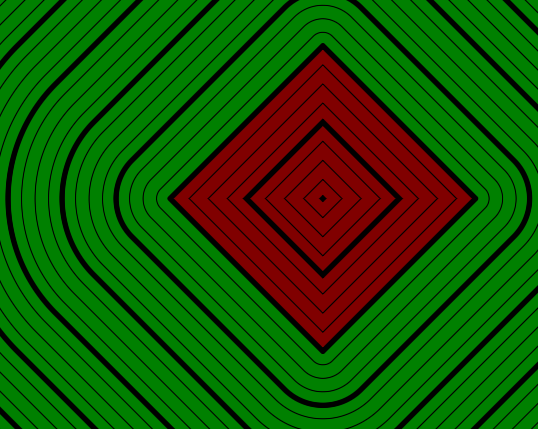## Source

### Library

``````#ifndef SDF_2D
#define SDF_2D

//transforms

float2 rotate(float2 samplePosition, float rotation){
const float PI = 3.14159;
float angle = rotation * PI * 2 * -1;
float sine, cosine;
sincos(angle, sine, cosine);
return float2(cosine * samplePosition.x + sine * samplePosition.y, cosine * samplePosition.y - sine * samplePosition.x);
}

float2 translate(float2 samplePosition, float2 offset){
//move samplepoint in the opposite direction that we want to move shapes in
return samplePosition - offset;
}

float2 scale(float2 samplePosition, float scale){
return samplePosition / scale;
}

//combinations

///basic
float merge(float shape1, float shape2){
return min(shape1, shape2);
}

float intersect(float shape1, float shape2){
return max(shape1, shape2);
}

float subtract(float base, float subtraction){
return intersect(base, -subtraction);
}

float interpolate(float shape1, float shape2, float amount){
return lerp(shape1, shape2, amount);
}

/// round
float round_merge(float shape1, float shape2, float radius){
float2 intersectionSpace = float2(shape1 - radius, shape2 - radius);
intersectionSpace = min(intersectionSpace, 0);
float insideDistance = -length(intersectionSpace);
float simpleUnion = merge(shape1, shape2);
float outsideDistance = max(simpleUnion, radius);
return  insideDistance + outsideDistance;
}

float round_intersect(float shape1, float shape2, float radius){
float2 intersectionSpace = float2(shape1 + radius, shape2 + radius);
intersectionSpace = max(intersectionSpace, 0);
float outsideDistance = length(intersectionSpace);
float simpleIntersection = intersect(shape1, shape2);
float insideDistance = min(simpleIntersection, -radius);
return outsideDistance + insideDistance;
}

float round_subtract(float base, float subtraction, float radius){
return round_intersect(base, -subtraction, radius);
}

///champfer
float champfer_merge(float shape1, float shape2, float champferSize){
const float SQRT_05 = 0.70710678118;
float simpleMerge = merge(shape1, shape2);
float champfer = (shape1 + shape2) * SQRT_05;
champfer = champfer - champferSize;
return merge(simpleMerge, champfer);
}

float champfer_intersect(float shape1, float shape2, float champferSize){
const float SQRT_05 = 0.70710678118;
float simpleIntersect = intersect(shape1, shape2);
float champfer = (shape1 + shape2) * SQRT_05;
champfer = champfer + champferSize;
return intersect(simpleIntersect, champfer);
}

float champfer_subtract(float base, float subtraction, float champferSize){
return champfer_intersect(base, -subtraction, champferSize);
}

/// round border intersection
float round_border(float shape1, float shape2, float radius){
float2 position = float2(shape1, shape2);
float distanceFromBorderIntersection = length(position);
return distanceFromBorderIntersection - radius;
}

float groove_border(float base, float groove, float width, float depth){
float circleBorder = abs(groove) - width;
float grooveShape = subtract(circleBorder, base + depth);
return subtract(base, grooveShape);
}

//shapes

float circle(float2 samplePosition, float radius){
//get distance from center and grow it according to radius
return length(samplePosition) - radius;
}

float rectangle(float2 samplePosition, float2 halfSize){
float2 componentWiseEdgeDistance = abs(samplePosition) - halfSize;
float outsideDistance = length(max(componentWiseEdgeDistance, 0));
float insideDistance = min(max(componentWiseEdgeDistance.x, componentWiseEdgeDistance.y), 0);
return outsideDistance + insideDistance;
}

#endif
``````

### Shader base

``````Shader "Tutorial/035_2D_SDF_Combinations/Round"{
Properties{
_InsideColor("Inside Color", Color) = (.5, 0, 0, 1)
_OutsideColor("Outside Color", Color) = (0, .5, 0, 1)

_LineDistance("Mayor Line Distance", Range(0, 2)) = 1
_LineThickness("Mayor Line Thickness", Range(0, 0.1)) = 0.05

[IntRange]_SubLines("Lines between major lines", Range(1, 10)) = 4
_SubLineThickness("Thickness of inbetween lines", Range(0, 0.05)) = 0.01
}

SubShader{
//the material is completely non-transparent and is rendered at the same time as the other opaque geometry
Tags{ "RenderType"="Opaque" "Queue"="Geometry"}

Pass{
CGPROGRAM

#include "UnityCG.cginc"
#include "2D_SDF.cginc"

#pragma vertex vert
#pragma fragment frag

struct appdata{
float4 vertex : POSITION;
};

struct v2f{
float4 position : SV_POSITION;
float4 worldPos : TEXCOORD0;
};

v2f vert(appdata v){
v2f o;
//calculate the position in clip space to render the object
o.position = UnityObjectToClipPos(v.vertex);
//calculate world position of vertex
o.worldPos = mul(unity_ObjectToWorld, v.vertex);
return o;
}

float scene(float2 position) {
const float PI = 3.14159;

float2 squarePosition = position;
squarePosition = translate(squarePosition, float2(1, 0));
squarePosition = rotate(squarePosition, .125);
float squareShape = rectangle(squarePosition, float2(2, 2));

float2 circlePosition = position;
circlePosition = translate(circlePosition, float2(-1.5, 0));
float circleShape = circle(circlePosition, 2.5);

float combination = /* combination calculation here */;

return combination;
}

float4 _InsideColor;
float4 _OutsideColor;

float _LineDistance;
float _LineThickness;

float _SubLines;
float _SubLineThickness;

fixed4 frag(v2f i) : SV_TARGET{
float dist = scene(i.worldPos.xz);
fixed4 col = lerp(_InsideColor, _OutsideColor, step(0, dist));

float distanceChange = fwidth(dist) * 0.5;
float majorLineDistance = abs(frac(dist / _LineDistance + 0.5) - 0.5) * _LineDistance;
float majorLines = smoothstep(_LineThickness - distanceChange, _LineThickness + distanceChange, majorLineDistance);

float distanceBetweenSubLines = _LineDistance / _SubLines;
float subLineDistance = abs(frac(dist / distanceBetweenSubLines + 0.5) - 0.5) * distanceBetweenSubLines;
float subLines = smoothstep(_SubLineThickness - distanceChange, _SubLineThickness + distanceChange, subLineDistance);

return col * majorLines * subLines;
}

ENDCG
}
}
FallBack "Standard" //fallback adds a shadow pass so we get shadows on other objects
}
``````

You can also find me on twitter at @totallyRonja. If you liked my tutorial and want to support me you can do that on Patreon (patreon.com/RonjaTutorials) or Ko-Fi (ko-fi.com/RonjaTutorials).